Courses

# Layout Plant And Control Chart - MCQ Test 1

## 20 Questions MCQ Test Mock Test Series - Mechanical Engineering (ME) for GATE 2020 | Layout Plant And Control Chart - MCQ Test 1

Description
This mock test of Layout Plant And Control Chart - MCQ Test 1 for Mechanical Engineering helps you for every Mechanical Engineering entrance exam. This contains 20 Multiple Choice Questions for Mechanical Engineering Layout Plant And Control Chart - MCQ Test 1 (mcq) to study with solutions a complete question bank. The solved questions answers in this Layout Plant And Control Chart - MCQ Test 1 quiz give you a good mix of easy questions and tough questions. Mechanical Engineering students definitely take this Layout Plant And Control Chart - MCQ Test 1 exercise for a better result in the exam. You can find other Layout Plant And Control Chart - MCQ Test 1 extra questions, long questions & short questions for Mechanical Engineering on EduRev as well by searching above.
QUESTION: 1

### The manufacturing area of a plat is divided into four quadrants. Four machines have to be located, one in each quadrant. The total number of possible layouts is:

Solution:

In quadrant I, we can locate any one of the four machines (i.e.) we can allocate quadrant I in 4 ways. Thereafter quadrant II in 3 ways, thereafter quadrant III in 2 ways. No further choice for quadrant IV.
∴ Total number of possible layouts
= 4 × 3 × 2 = 24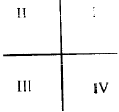QUESTION: 2

### Statistical qua ntity con trol was developed by: [GATE-1995]

Solution:

Dr. Waher Shewhart an American scientist during World War-II.

QUESTION: 3

### Vehicle manufacturing assembly line is an example of:

Solution:
QUESTION: 4

In a weaving operation, the parameter to be controlled is the number of defects per 10 square yards of material. Control chart appropriate for, his task is:

[GATE-1998]

Solution:
QUESTION: 5

The symbol used for Transport in work study is:

Solution:
QUESTION: 6

Match List-I (Quality control concepts) with List-II (Quality control techniques) and select the correct answer using the codes given below the lists: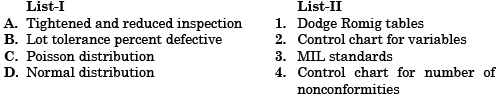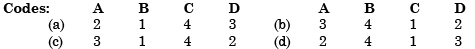Solution:
QUESTION: 7

An assembly activity is represented on an Operation Process Chart by the symbol

Solution:
QUESTION: 8

Quality control chart for averages was maintained for a dimension of the product. After the control was established, it was found that the standard deviation (σ) of the process was 1.00 mm The dimension of the part is 70 ± 2.5 mm. Parts above 72.5 mm can be reworked but parts below 67.5 mm have to be scrapped. What should be the setting of the process to ensure production of no scrap and to minimize the rework?

Solution:

Standard deviation from mean = 0.5 mm
Therefore for no scrap D = 70 + 0.5 = 70.5 mm

QUESTION: 9

Consider the following statements regarding plant location and plant layout:

1. Qualitative factor analysis is a method of evaluating a potentiallocation without applying quantitative values to the decision criteria.
2. The three determinants of the type of layout are type of product, type of process and the volume of production.
3. An appliance manufacturing plant where products are made on assembly lines would be classified as job shop type of layout.

Which of these statements is/are correct?

Solution:
QUESTION: 10

The graph shows the results of various quality levels for a component

Consider the following statements:

1. Curve A shows the variation of value of component
2. Curve B shows the variation of cost of the component
3. Graph is called as fish bone diagram
4. The preferred level of quality is given by line CC
5. The preferred level of quality is given by line DD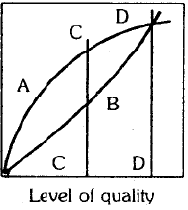Which of the above statements are correct?

Solution:
QUESTION: 11

Match List-I (Object) with List-II (Tool) and select the correct answer: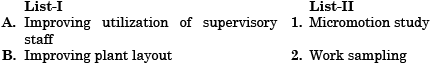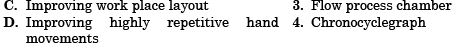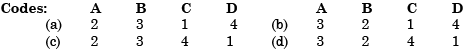Solution:
QUESTION: 12

Match List-I (Scientist) with List-II (Research work) and select the correct answer using the codes given below the lists: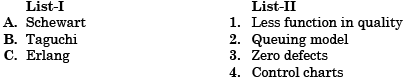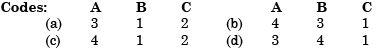Solution:
QUESTION: 13

Which of the following charts are used for plant layout design?

1. Operation process chart
2. Man machine chart
3. Correlation chart
4. Travel chart

Select the correct answer using the codes given below:

Solution:
QUESTION: 14

Assertion (A): In case of Control Chart for fraction rejected (pchart), binomial distribution is used.

Reason (R): In binomial distribution probability of the event varies with each draw.

Solution:
QUESTION: 15

Which one of the following is correct?

Production planning and control functions are extremely complex in:

Solution:

Production, planning and control function are extremely complex in batch production shop producing a batch at irregular intervals.

QUESTION: 16

Assertion (A): In case of Control Charts for variables, the averages of sub-groups of readings are plotted instead of plotting individual readings.

Reason (R): It has been proved through experiments that averages will form normal distribution

Solution:
QUESTION: 17

Which one of the following combinations is valid for product layout?

Solution:
QUESTION: 18

Assertion (A): In case of control charts for variables, the average of readings of a subgroup of four and more is plotted rather than the individual readings.

Reason (R): Plotting of individual readings needs a lot of time and effort.

Solution:
QUESTION: 19

The type of layout suitable for use of the concept, principles and approaches of ‘group technology’ is:

Solution:
QUESTION: 20

The span of control refers to the

Solution: